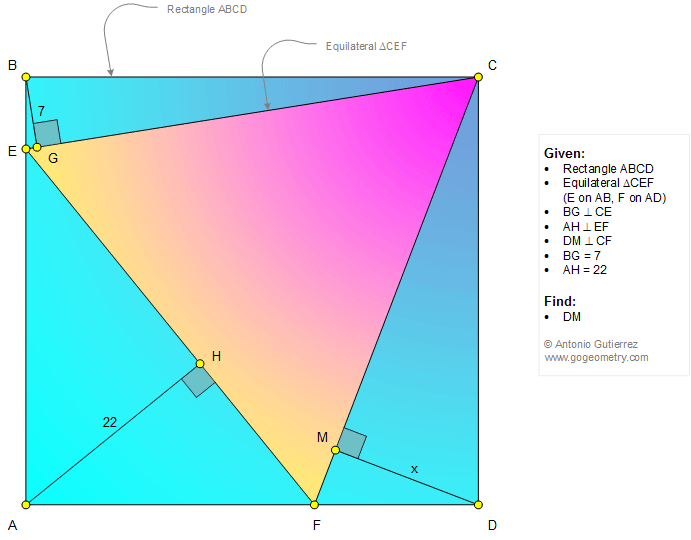#Geometry Problem 1318: Rectangle, Equilateral Triangle, Distances, Perpendicular, Measurement

 < PREVIOUS PROBLEM  |  NEXT PROBLEM > The figure shows a rectangle ABCD and an equilateral triangle CEF ( E on AB and F on AD). The distances from B and A to CE and EF are 7 and 22, respectively. Find the distance from D to CF.See also: Sketch of problem 1318 using mobile apps Kaleidoscope of problem 1318 using iPad Apps

 Home | Geometry | Problems | All Problems | Open Problems | Visual Index | 1311-1320 | Triangle | Equilateral Triangle | Rectangle | Perpendicular line | View or Post a solution | by Antonio Gutierrez